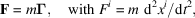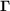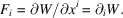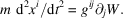International
Tables for
Crystallography
Volume D
Physical properties of crystals
Edited by A. Authier

International Tables for Crystallography (2006). Vol. D, ch. 1.1, p. 9

## Section 1.1.3.6.3. Examples of the use in physics of different representations of the same quantity

A. Authiera*

aInstitut de Minéralogie et de la Physique des Milieux Condensés, Bâtiment 7, 140 rue de Lourmel, 75015 Paris, France
Correspondence e-mail: aauthier@wanadoo.fr

#### 1.1.3.6.3. Examples of the use in physics of different representations of the same quantity

| top | pdf |

Let us consider, for example, the force, F, which is a tensor quantity (tensor of rank 1). One can define it:

 (i) by the fundamental law of dynamics:where m is the mass andis the acceleration. The force appears here in a contravariant form. (ii) as the derivative of the energy, W:The force appears here in covariant form. In effect, we shall see in Section 1.1.3.8.1that to form a derivative with respect to a variable contravariant augments the covariance by unity. The general expression of the law of dynamics is therefore written with the energy as follows: